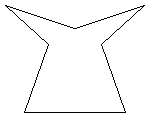#### You may also like### Floored

A floor is covered by a tessellation of equilateral triangles, each having three equal arcs inside it. What proportion of the area of the tessellation is shaded?### Getting an Angle

How can you make an angle of 60 degrees by folding a sheet of paper twice?### Arclets Explained

This article gives an wonderful insight into students working on the Arclets problem that first appeared in the Sept 2002 edition of the NRICH website.

# Heptagon Has

##### Age 11 to 14 Short Challenge Level:

It can possess all five properties.The description does not say that heptagon has to be convex, i.e. all of its interior angles need not be less than $180^{\circ}$. Since $(2 \times 7 - 4) \times 90 = 900$, the interior angles of all heptagons total $900^{\circ}$. The creation of a heptagon with all the given conditions is possible as the diagram shows. Notice that four of the interior angles are acute and the other three are reflex angles.

This problem is taken from the UKMT Mathematical Challenges.
You can find more short problems, arranged by curriculum topic, in our short problems collection.